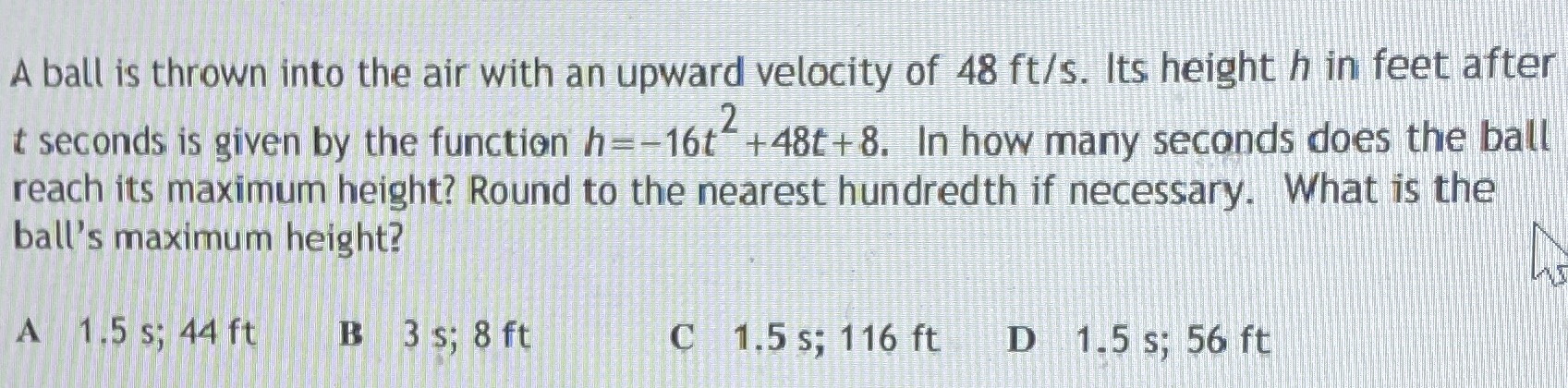### ¿Todavía tienes preguntas de matemáticas?

Pregunte a nuestros tutores expertos
Algebra
PreguntaA ball is thrown into the air with an upward velocity of $$48 ft / s$$ . Its height $$h$$ in feet after

$$t$$ seconds is given by the function $$h = - 16 t ^ { 2 } + 48 t + 8$$ . In how many seconds does the ball reach its maximum height? Round to the nearest hundredth if necessary. What is the ball's maximum height?

$$1.5 s ; 44 ft$$ B $$3 s ; 8 ft$$ C $$1.5 s ; 116 ft$$ D $$1.5 s ; 56 ft$$

$$1.5 s ; 44 ft$$